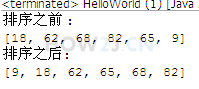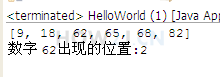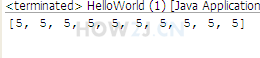# Java自学-数组 Arrays

2019/08/26 14:46

## java.util.Arrays类常用方法

Arrays是针对数组的工具类，可以进行 排序，查找，复制填充等功能。 大大提高了开发人员的工作效率。

import java.util.Arrays;

public class HelloWorld {
public static void main(String[] args) {
int a[] = new int[] { 18, 62, 68, 82, 65, 9 };

// copyOfRange(int[] original, int from, int to)
// 第一个参数表示源数组
// 第二个参数表示开始位置(取得到)
// 第三个参数表示结束位置(取不到)
int[] b = Arrays.copyOfRange(a, 0, 3);

for (int i = 0; i < b.length; i++) {
System.out.print(b[i] + " ");
}

}
}


import java.util.Arrays;

public class HelloWorld {
public static void main(String[] args) {
int a[] = new int[] { 18, 62, 68, 82, 65, 9 };
String content = Arrays.toString(a);
System.out.println(content);

}
}import java.util.Arrays;

public class HelloWorld {
public static void main(String[] args) {
int a[] = new int[] { 18, 62, 68, 82, 65, 9 };
System.out.println("排序之前 :");
System.out.println(Arrays.toString(a));
Arrays.sort(a);
System.out.println("排序之后:");
System.out.println(Arrays.toString(a));

}
}import java.util.Arrays;

public class HelloWorld {
public static void main(String[] args) {
int a[] = new int[] { 18, 62, 68, 82, 65, 9 };

Arrays.sort(a);

System.out.println(Arrays.toString(a));
//使用binarySearch之前，必须先使用sort进行排序
System.out.println("数字 62出现的位置:"+Arrays.binarySearch(a, 62));
}
}


import java.util.Arrays;

public class HelloWorld {
public static void main(String[] args) {
int a[] = new int[] { 18, 62, 68, 82, 65, 9 };
int b[] = new int[] { 18, 62, 68, 82, 65, 8 };

System.out.println(Arrays.equals(a, b));
}
}import java.util.Arrays;

public class HelloWorld {
public static void main(String[] args) {
int a[] = new int;

Arrays.fill(a, 5);

System.out.println(Arrays.toString(a));

}
}


(首先定义一个5X8的二维数组，然后使用随机数填充满。 借助Arrays的方法对二维数组进行排序。 参考思路： 先把二维数组使用System.arraycopy进行数组复制到一个一维数组 然后使用sort进行排序 最后再复制回到二维数组。)

0
0 收藏

### 作者的其它热门文章0 评论
0 收藏
0